AP Calculus BC Review: Series Convergence

A series is the sum of infinitely many terms. But how can you add up an infinite number of things? Well, it turns out that sometimes we can do exactly that! But first we’ll need to understand the concepts of series convergence and divergence.Infinite series convergence may seem mysterious at first, but it’s not the work of aliens…. so far as I know.

Working with Series

Typically, a series is expressed either by writing out a few terms in order to establish a pattern, or by using sigma notation.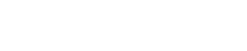Here, an is the general term for the series.

Check out the following article for more explanation as well as examples: AP Calculus BC Review: Series Fundamentals.

Partial Sums

Any time that infinity is involved, we have to be extra careful with our definitions. So how do you add up infinitely many items? One item at a time! This is a job for partial sums.

A partial sum is something like a running total for a series.

Let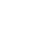be a series.

The kth partial sum of the series is: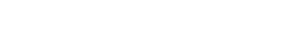In other words, the kth partial sum is the sum of the first k terms of the series.

The first four partial sums of a series are:Now, the useful thing about partial sums is that they form a sequence,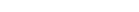And then we define series convergence in terms of the convergence of this sequence of partial sums.

Series Convergence and Divergence — Definitions

A series Σan converges to a sum S if and only if the sequence of partial sums converges to S. That is, a series converges if the following limit exists: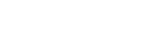Otherwise, if the limit of sk (as k → ∞) is infinite or fails to exist, then the series diverges.

Ways That a Series Could Diverge

A series may diverge in three different ways:

1. The series diverges to infinity (∞) if the partial sums increase without bound. That is, sk → ∞.
2. The series diverges to negative infinity (-∞) if the partial sums decrease without bound. That is, sk → -∞.
3. If the limit of partial sums does not exists and is not ∞ or -∞, then we just say that the series diverges. This case occurs whenever the sequence of partial sums oscillates among multiple values or jumps around randomly.

Limit Test for Divergence

There is a quick and easy test that can be used to show that a series diverges. (However, it can’t be used to show a series converges.)

Limit Divergence Test: If the limit of the general term of a series is not equal to 0, then the series diverges.

That is, if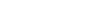, then the series Σan diverges.

Unfortunately, if the limit does turn out to be zero, then the test is inconclusive. Then you’d have to use additional convergence tests to figure out series convergence or divergence.

Let’s take a look at a few examples.

Using the Limit Divergence Test

Which of the following series must diverge according to the Divergence Test?Solutions

Remember, for this question, we’re only allowed to use the Divergence Test.

(a) As n → ∞, the values of 2n also approach ∞. The bottom line is that the limit of 2n is certainly not equal to 0.

Therefore, series (a) diverges.

(b)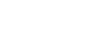Here, the answer is not clear. A limit of 0 does not automatically mean that the series will not diverge. So the Limit Divergence Test alone cannot say anything more about series convergence or divergence here.

In fact, we will prove that this series does converge by other methods.

(c) Again, the Divergence Test is inconclusive, because 1/n → 0 as n → ∞.

Now this series actually diverges. It’s called the Harmonic Series, and it’s just the sum of all of the reciprocals of the natural numbers.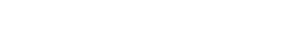We’ll need another method to decide for sure that the Harmonic Series diverges. It’s far from obvious!

(d)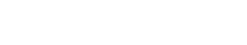With a nonzero limit, the Divergence Test conclusively states that this series must diverge.

Geometric Series

Let’s take a closer look at Examples (a) and (b). Both of these are geometric series.

A geometric series is the sum of the powers of a constant base.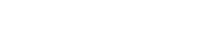There is a straightforward test to decide whether any geometric series converges or diverges.

• If |r| < 1, then the series converges.
• If |r| ≥ 1, then the series diverges.

Furthermore, whenever the series does converge, there is a simple formula to find its sum: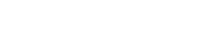Going back to Example (a), Σ 2n is a geometric series with base r = 2. Because |r| = 2 ≥ 1, this series diverges. (Of course, we knew that already from the Limit Divergence Test, but it’s nice to see confirmation from other sources.)

The series in Example (b) is also geometric, but you have to write it in an equivalent form to see why.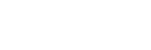Here, the base is 1/2, and since |r| = 1/2 < 1, now we know that this series does indeed converge. We can even find the value of the sum!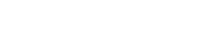Another way to state this result is to say that: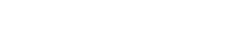So, all the fractional parts, 1/2, 1/4, /18, etc. can fit side-by-side into a single unit interval of the number line!A ruler showing that the sum 1/2 + 1/4 + 1/8 + 1/16 + …. = 1.

Check out the following post for more on geometric series: AP Calculus BC Review: Geometric Series.

Integral Test

Finally, let’s talk about a convergence test that may help out in certain specialized situations.

Integral Test: Suppose that we have a series Σan.

If all of the following are true,

1. the general term an is positive for every n,
2. the general terms decrease as n increases,
3. and the formula an = f(n) is continuous,

then the series converges if and only if the improper integral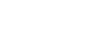converges. Here, we usually take the lower limit a to be the same as the lower limit of the sum.

Now, what’s really nice about the integral test is that it can test for both convergence and divergence.

Let’s see how it works on the Harmonic Series.

The general term, an = 1/n, is positive, decreasing, and continuous (for positive n, anyway).

Next, set up the integral. Don’t forget to change your n into x in the formula for the general term.Because the integral diverges (to ∞), the series also diverges.Improve your SAT or ACT score, guaranteed. Start your 1 Week Free Trial of Magoosh SAT Prep or your 1 Week Free Trial of Magoosh ACT Prep today!Author

•Shaun earned his Ph. D. in mathematics from The Ohio State University in 2008 (Go Bucks!!). He received his BA in Mathematics with a minor in computer science from Oberlin College in 2002. In addition, Shaun earned a B. Mus. from the Oberlin Conservatory in the same year, with a major in music composition. Shaun still loves music -- almost as much as math! -- and he (thinks he) can play piano, guitar, and bass. Shaun has taught and tutored students in mathematics for about a decade, and hopes his experience can help you to succeed!